Latest Banking jobs   »

# Reasoning Quiz For Bank of Baroda AO 2023-25th April

Direction (1-3): Study the following information carefully to answer the given questions:
P, Q, R, S, U, V and T are the members of a family. There are three generation in this family. P is mother-in-law of T. V is sister of S who is nephew of R. U is granddaughter of Q. T is not married to V. T is a married woman. R is not sibling of P.

Q1. Who among the following is niece of V?
(a) U
(b) Q
(c) R
(d) T
(e) None of these

Q2. How R is related to V?
(a) Niece
(b) Father
(c) Aunt
(d) Uncle
(e) Cannot be determine

Q3. Who among the following is mother of U?
(a) V
(b) R
(c) P
(d) T
(e) None of these

Q4. A person starts walking towards point Y which in North direction of starting point. From point Y, he starts walking in west direction then he takes right turn to reach at point W. From point W, he takes a right turn and stop at point J. Find in which direction person is facing now?
(a) North
(b) South
(c) East
(d) West
(e) None of these

Q5. Point H is 5m west of J. Point J is 10m south of point T. Point T is 12m west of G. Point G is 6m north of Point D. Point D is 15m east of Point Y. What is the shortest distance between Point J and Point Y?
(a) 10m
(b) 4m
(c) 9m
(d) 7m
(e) None of these

Directions (6-10): In each of the questions below some statements are given followed by some Conclusions. You have to take the given statements to be true even, if they seem to be at variance from commonly known facts. Read all the conclusions and then decide which of the given conclusions logically follows from the given statements disregarding commonly known facts.
(a) If only conclusion I follows.
(b) If only conclusion II follows.
(c) If either conclusion I or II follows.
(d) If neither conclusion I nor II follows.
(e) If both conclusions I and II follow.

Q6. Statements: Only few games are apple
All mango are orange
No apple is Orange
Conclusions: I: All games can never be orange
II: Some mango are not games

Q7. Statements: Only grapes are papaya.
Some grapes are mango.
No mango is litchi.
Conclusions: I: Some papaya is not litchi
II: Some papaya is mango

Q8. Statements: Some Word is sentence.
All sentences are language.
Conclusions: I. All radio is language is possibility.
II. Some Language are not Radio.

Q9. Statements: Some North are south
Only a few South are west.
Only a few west are east
Conclusions: I. All west can be east
II. All north can be south

Q10. Statements: All word are juice.
Some juice are shakes
Some word are pure.
Conclusions: I. Some Shakes are Pure
II. No Pure is shakes

Direction (11-15): Read the following arrangement carefully to answer the following questions.

K Z 3 + T % & I H N ! 8 \$ J Q 1 L M * @ Y R 2 4 # D F =

Q11. How many such symbols are there in the above arrangement each of which is either immediately followed by a number or immediately preceded by a letter, but not both?
(a) None
(b) One
(c) Two
(d) Three
(e) More than three

Q12. Four of the following five are alike in a certain way. Which one does not belong to the group?
(a) 3 Z D F
(b) % T 2 4
(c) K T = 4
(d) % I 2 Y
(e) + # @ Q

Q13. If 3 Z T is related to & H % in the same way, Q J L is to ____?
(a) Y R *
(b) * Y M
(c) @ * R
(d) @ Y L
(e) None of these

Q14. How many such numbers are there, each of which is either immediately followed by a symbol or immediately preceded by a letter, but not both?
(a) None
(b) One
(c) Two
(d) Three
(e) More than three

Q15. How many such letters are there, each of which is either immediately followed by a number or immediately preceded by a symbol but not both?
(a) None
(b) One
(c) Two
(d) Three
(e) More than three

Solutions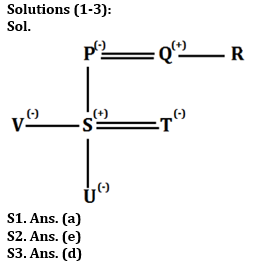S4. Ans. (c)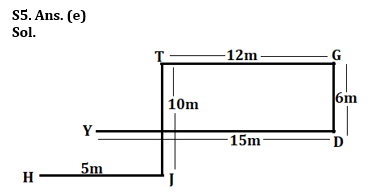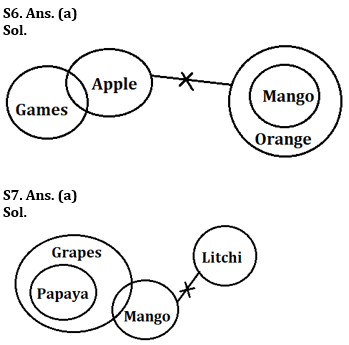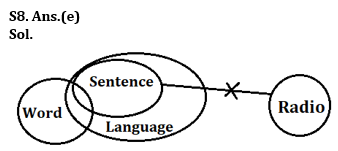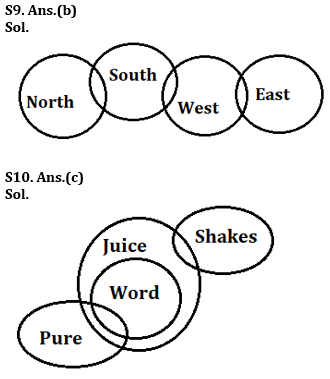Solution (11-15):
S11. Ans. (d)
Sol. K Z 3 + T % & I H N ! 8 \$ J Q 1 L M * @ Y R 2 4 # D F =
So, there are three symbols.

S12. Ans. (e)
Sol. In all other groups, the 3rd and 4th elements occupy the same positions from the right in the given arrangement as the first and second elements respectively from the left end.

S13. Ans. (b)
Sol. In each pair, the 1st, 2nd and 3rd elements of the 1st term move 4, 7 and 1 steps forward respectively to give the corresponding elements of the 2nd term.

S14. Ans. (e)
Sol. Series – K Z 3 + T % & I H N ! 8 \$ J Q 1 L M * @ Y R 2 4 # D F =
So, there are four numbers.

S15. Ans. (e)
Sol. Series – K Z 3 + T % & I H N ! 8 \$ J Q 1 L M * @ Y R 2 4 # D F =
So, there are eight alphabets.## FAQs

### What is the selection process of Bank of Baroda AO 2023?

The selection process of Bank of Baroda AO consists of Online exam and Interview

#### Congratulations!Union Budget 2023-24: Free PDF